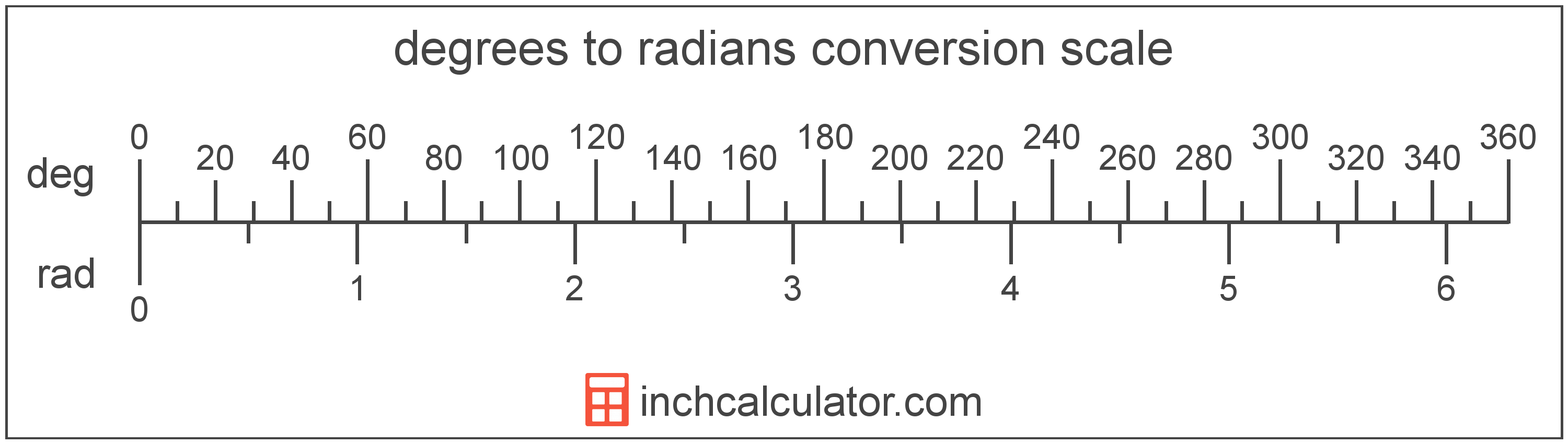Enter the angle in radians below to get the value converted to degrees. The calculator supports values containing decimals, fractions, and π: (π/2, 1/2π, etc)

Results in Degrees:2 rad = 114° 35′ 29.61″
2 rad = 360 / π

Do you want to convert degrees to radians?

## How to Convert Radians to Degrees

To convert a measurement in radians to a measurement in degrees, you need to use a conversion formula. Since pi radians are equal to 180°, the following conversion formula is preferred in mathematics for its accuracy and convenience.

degrees = radians × 180 / π

In other words, the angle in degrees is equal to the radians times 180 divided by pi.

To use this formula, start by substituting the angle, in radians, into the formula. Then, move the radians to the top of the fraction, and finally simplify the fraction and evaluate.

For example, let's convert 5 radians to degrees using the preferred formula.
degrees = 5 rad × 180 / π
degrees = 5 rad × 180 / π
degrees = 900 / π

### Alternate Radian to Degree Formula

If you want to convert radians to degrees without using pi, multiply the angle by the following conversion ratio: 57.29578 degrees/radian.

Since one radian is equal to 57.29578 degrees, you can use this simple formula to convert:

The angle in degrees is equal to the angle in radians multiplied by 57.29578.

For example, here's how to convert 5 radians to degrees using this formula.
degrees = (5 rad × 57.29578) = 286.478898°### How Many Degrees Are in a Radian?

There are exactly 180/π degrees in a radian.

1 rad = 180° / π

Without using pi, there are approximately 57.29578 degrees in a radian.

A radian is the measurement of angle equal to the length of an arc divided by the radius of the circle or arc. 1 radian is equal to 180/π degrees, or about 57.29578°. There are about 6.28318 radians in a circle.

The radian is the SI derived unit for angle in the metric system. Radians can be abbreviated as rad, and are also sometimes abbreviated as c, r, or R. For example, 1 radian can be written as 1 rad, 1 c, 1 r, or 1 R.

Radians are often expressed using their definition. The formula to find an angle in radians is θ = s/r, where the angle in radians θ is equal to the arc length s divided by the radius r. Thus, radians may also be expressed as the formula of arc length over the radius.

Radians are also considered to be a "unitless" unit. That is, when multiplying or dividing by radians, the result does not include radians as part of the final units.

For example, when determining the length of an arc for a given angle, we use the formula above, rearranged to be s = θr. If θ is in radians and r is in meters, then the units of s will be meters, not radian-meters. If θ were in degrees, however, then s would have units of degree-meters.

## What Is a Degree?

A degree is a measure of angle equal to 1/360th of a revolution, or circle. The number 360 has 24 divisors, making it a fairly easy number to work with. There are also 360 days in the Persian calendar year, and many theorize that early astronomers used 1 degree per day.

The degree is an SI accepted unit for angle for use with the metric system. A degree is sometimes also referred to as a degree of arc, arc degree, or arcdegree. Degrees can be abbreviated as °, and are also sometimes abbreviated as deg. For example, 1 degree can be written as 1° or 1 deg.

Degrees can also be expressed using arcminutes and arcseconds as an alternative to using the decimal form. Arcminutes and arcseconds are expressed using the prime (′) and double-prime (″) characters, respectively, although a single-quote and double-quote are often used for convenience.

One arcminute is equal to 1/60th of a degree, and one arcsecond is equal to 1/60th of an arcminute.

Protractors are commonly used to measure angles in degrees. They are semi-circle or full-circle devices with degree markings allowing a user to measure an angle in degrees. Learn more about how to use a protractor or download a printable protractor.

## Radian to Degree Conversion Table

Radian values as a mathematical expression converted to a decimal value and degree value.